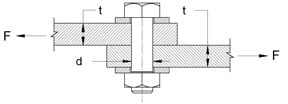Bolt or Pin In Single Shear Equation and Calculator

Bolt or Pin In Single Shear Equation and Calculator. Keep units consistant when performing calculations.Bolt or Pin Single Shear Stress Applied Force F (N, lbs) = Bolt/Pin Diameter d (mm, in) = Plate Thickness t (mm, in) = Ultimate Stress (N/mm2, lbs/in2) = Factor of Safety = Results Section Area of Bolt/Pin (mm2, in2) = Shear Stress ave Bolt/Pin (N/mm2, lbs/in2) = Bearing Area Stress Bt (N/mm2, lbs/in2 = Allowable Stress (N/mm2, lbs/in2) =

Shear Stress Equation Single Shear

Shear Stress Average = Applied Force / Area
or
Shear Stress ave.= F/( π r 2)
or
Shear Stress ave.= 4F/( π d 2)

Where:
Shear Stress ave = ( N/mm2, lbs/in2)
F = Applied Force (N, Lbs)
π = pi or (3.14157)
d = Diameter (mm, in.)

Bearing Area Stress Equation for Plate and Bolt or Pin

Bt= F / (t d)

Where:
Bt = Bearing Area Stress Area ( N/mm2, lbs/in2)
F = Applied Force (N, lbs)
t = Thickness (mm, in)
d = Diameter (mm, in)

Factor of Safety

Factor of Safety = F.S = ultimate stress / allowable stress

Therefore:

allowable stress = ultimate stress / F.S.

Related:Membership Register | LoginHomeEngineering Book StoreEngineering ForumExcel App. DownloadsOnline Books & ManualsEngineering NewsEngineering VideosEngineering CalculatorsEngineering ToolboxGD&T Training Geometric Dimensioning TolerancingDFM DFA TrainingTraining Online EngineeringAdvertising CenterCopyright Notice